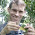## Thursday, March 15, 2012

### Contrasts regression with multifurcations

An R phylogenetics user just emailed me about a post I wrote (archived here) describing how to resolve multifurcations before calculating contrasts for PIC regression. I just wanted to take a couple of moments to elaborate on this issue.

The contrasts algorithm (Felsenstein 1985) requires a fully dichotomous (i.e. fully bifurcating) phylogenetic tree with branch lengths. By computing the differences between tip & node states, the contrasts algorithm creates a transformed dataset which (under a Brownian model of evolution) is free from phylogenetic dependence.

However, if your phylogeny is not fully dichotomous, it can be rendered dichotomous by arbitrarily resolving all polytomies through the addition of one or more internal branches of length 0.0; after which contrasts can be calculated as normal. Since every trifurcation can be resolved in at least three ways (and higher order multifurcations in even more different ways), we may have many options in how we choose to resolve our non-dichotomous tree. For instance, if our tree is fully bifurcating except at two nodes which are each trifurcations, we have 3 × 3 = 9 different ways of rendering our tree dichotomous via the addition of internal zero length branches.

Luckily, it turns out not to matter how we resolve the tree: statistical analysis of the contrasts transformed data is unaffected. To prove this to ourselves, let's look at two different examples:

> require(phytools)
> # first let's create a random tree with polytomies
> tree<-rtree(n=100)
> # pick nodes to collapse
> nodes<-100+sample(2:tree\$Nnode)[1:30]
> # match the nodes to tree\$edge & set to zero
> tree\$edge.length[apply(apply(as.matrix(nodes),1,"==", as.matrix(tree\$edge[,2])),2,which)]<-0
> # collapse 0 length branches to multifurcations
> tree<-di2multi(tree)
> plotTree(tree,pts=F,ftype="off")Close inspection of this tree reveals that it has many multifurcations, and this is corroborated by the fact that the tree can be seen to have 30 fewer nodes (just as we'd hoped) than the number expected for a fully dichotomous 100 taxon tree:

> tree\$Nnode
 69

The next thing we'll do is simulate under an arbitrary linear model, here y = 1.5 + 0.75x + ε, but we will do so in such a way so that the data are phylogenetically autocorrelated:

> x<-fastBM(tree) # explanatory variable
> y<-1.5+0.75*x+fastBM(tree,sig2=0.2) # arbitrary linear model

OK, now let's resolve the tree randomly, compute contrasts, and fit a bivariate regression model to the contrasts:

> rt1<-multi2di(tree,random=TRUE)
> pic.x<-pic(x,rt1)
> pic.y<-pic(y,rt1)
> lm(pic.y~pic.x-1) # fits the regression model without an intercept

Call:
lm(formula = pic.y ~ pic.x - 1)

Coefficients:
pic.x
0.7761

To show that we get the same result from contrasts regression regardless of the random resolution that we use, let's try a different random resolution and fit the regression model again:

> rt2<-multi2di(tree,random=TRUE)
> pic.x<-pic(x,rt2)
> pic.y<-pic(y,rt2)
> lm(pic.y~pic.x-1)

Call:
lm(formula = pic.y ~ pic.x - 1)

Coefficients:
pic.x
0.7761

To assure ourselves that this is not just magical good luck at having obtained the same resolution in each case we can compute:

> all.equal.phylo(rt1,rt2)
 FALSE

and see that it is FALSE.

We have to avoid the following tempting shortcut:

> lm(pic(y,multi2di(tree))~pic(x,multi2di(tree))-1)

Call:
lm(formula = pic(y, multi2di(tree)) ~ pic(x, multi2di(tree)) -
1)

Coefficients:
pic(x, multi2di(tree))
-0.01451

or variants thereof, because in this case different random resolutions are used for x & y, which is not permitted. However, now knowing that the specific choice of resolution doesn't matter (so long as it is the same for dependent & independent variables), we can also see that the following will work:

> lm(pic(y,multi2di(tree,random=FALSE))~pic(x,multi2di(tree, random=FALSE))-1)

Call:
lm(formula = pic(y, multi2di(tree, random = FALSE)) ~ pic(x,
multi2di(tree, random = FALSE)) - 1)

Coefficients:
pic(x, multi2di(tree, random = FALSE))
0.7761

In this case, multi2di(...,random=FALSE) polytomies are not resolved randomly, but in the order they appear in the tree (which appears to be right-to-left in the input Newick string).

1.I have been trying to render my tree dichotomous to calculate the amount of phylogenetic signal usinf pagel's 1999 lamda but the program (r) crashes all the time. Any suggestions as to what is the problem?

1.You can estimate phylogenetic signal using lambda or K in phylosig for trees that are not fully bifurcating. If that does work - please feel free to email me your data and tree and I will troubleshoot. Liam

2.Hello,
I have used multi2di to render my tree dichotomous, but when I ask:is.binary.tree the R program return FALSE. Do you have any suggestion for me?

library(picante)